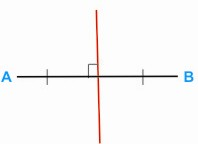HOME MATH DICTIONARY DOWNLOAD FEEDBACK DISCLAIMER
 Question: What is a Locus ? Answer: The path traced out by a moving point, which can be defined as the set of all points on a curve satisfying given conditions. The locus of points a fixed distance from a fixed point is a circle. The locus of a point equidistant from two fixed points is a straight line that perpendicularly bisects the line joining them. The locus of points a fixed distance from a line is two parallel lines running either side. For example,the locus of points which are the same distance from two fixed points A and B is the red line in the diagram. It is the perpendicular bisector of AB.